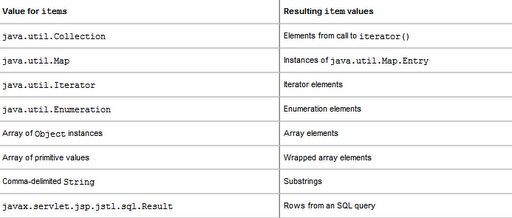# JSTL Size Of Map

JSTL Size Of Map explains about how to find the size of a map using JSTL Taglib

Consider the example here, a JSP page which contains a HashMap with lot of key value pairs in it and we need to find the size of this HashMap

We already seen how to find the size of a Collection using the JSTL fn:length function.

You can see it fn:length() JSTL Function .

Note

For iterating a Map, you can follow below tutorial

You can change JSTL forEach Map Iteration

###### Required Libraries

Following jar must be in classpath

1. jstl-1.2.jar

## Find JSTL Size Of Map

```// How to find size of a map using JSTL

<%@ taglib uri="http://java.sun.com/jsp/jstl/core" prefix="c"%>
<%@ page import="java.util.HashMap"%>
<%
HashMap<String,String> numMap = new HashMap<String,String>();
numMap.put("1","one");
numMap.put("2","two");
numMap.put("3","three");
request.setAttribute("numMap", numMap);
%>
<html>
<body>
Size is \${numMap.size()}
</body>
</html>
```
###### Output
Size is 3

Please check the table, You can iterate following table items using c:forEach tag